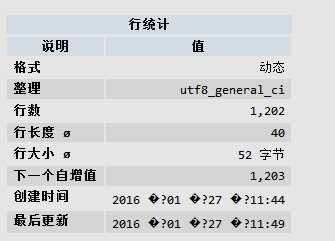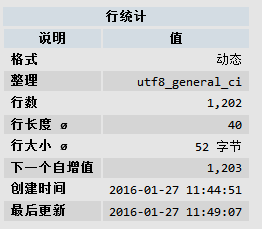```> 这个问题应该在php5.5.30 与 7.3.11中不再出现。或许，这是灵异问题。

### 现象：### 方案：

`        \$format = __('%B %d, %Y at %I:%M %p');`

`        \$format = '%Y-%m-%d %H:%M:%S';`### 更好的方案

```\$format = __('%B %d, %Y at %I:%M %p');
//patch for win
\$fs_ver=explode('.',PHP_VERSION);
if( \$fs_ver > 5 or (\$fs_ver = 5 && \$fs_ver >= 4) ){
if(strtoupper(substr(PHP_OS, 0, 3)) === 'WIN'){
\$format = '%Y-%m-%d %H:%M:%S';
}
}
```